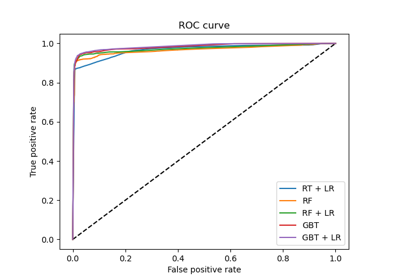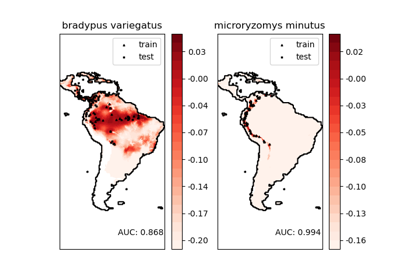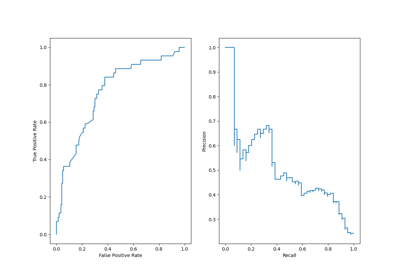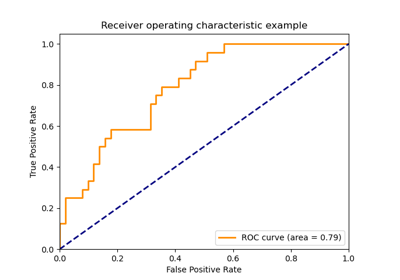# sklearn.metrics.roc_curve¶

sklearn.metrics.roc_curve(y_true, y_score, *, pos_label=None, sample_weight=None, drop_intermediate=True)[source]

Note: this implementation is restricted to the binary classification task.

Read more in the User Guide.

Parameters
y_truearray, shape = [n_samples]

True binary labels. If labels are not either {-1, 1} or {0, 1}, then pos_label should be explicitly given.

y_scorearray, shape = [n_samples]

Target scores, can either be probability estimates of the positive class, confidence values, or non-thresholded measure of decisions (as returned by “decision_function” on some classifiers).

pos_labelint or str, default=None

The label of the positive class. When pos_label=None, if y_true is in {-1, 1} or {0, 1}, pos_label is set to 1, otherwise an error will be raised.

sample_weightarray-like of shape (n_samples,), default=None

Sample weights.

drop_intermediateboolean, optional (default=True)

Whether to drop some suboptimal thresholds which would not appear on a plotted ROC curve. This is useful in order to create lighter ROC curves.

New in version 0.17: parameter drop_intermediate.

Returns
fprarray, shape = [>2]

Increasing false positive rates such that element i is the false positive rate of predictions with score >= thresholds[i].

tprarray, shape = [>2]

Increasing true positive rates such that element i is the true positive rate of predictions with score >= thresholds[i].

thresholdsarray, shape = [n_thresholds]

Decreasing thresholds on the decision function used to compute fpr and tpr. thresholds represents no instances being predicted and is arbitrarily set to max(y_score) + 1.

roc_auc_score

Compute the area under the ROC curve

Notes

Since the thresholds are sorted from low to high values, they are reversed upon returning them to ensure they correspond to both fpr and tpr, which are sorted in reversed order during their calculation.

References

1

Wikipedia entry for the Receiver operating characteristic

2

Fawcett T. An introduction to ROC analysis[J]. Pattern Recognition Letters, 2006, 27(8):861-874.

Examples

>>> import numpy as np
>>> from sklearn import metrics
>>> y = np.array([1, 1, 2, 2])
>>> scores = np.array([0.1, 0.4, 0.35, 0.8])
>>> fpr, tpr, thresholds = metrics.roc_curve(y, scores, pos_label=2)
>>> fpr
array([0. , 0. , 0.5, 0.5, 1. ])
>>> tpr
array([0. , 0.5, 0.5, 1. , 1. ])
>>> thresholds
array([1.8 , 0.8 , 0.4 , 0.35, 0.1 ])


## Examples using sklearn.metrics.roc_curve¶